Test: Dice- 2

# Test: Dice- 2

Test Description

## 10 Questions MCQ Test CSAT Preparation for UPSC CSE | Test: Dice- 2

Test: Dice- 2 for DSSSB TGT/PGT/PRT 2022 is part of CSAT Preparation for UPSC CSE preparation. The Test: Dice- 2 questions and answers have been prepared according to the DSSSB TGT/PGT/PRT exam syllabus.The Test: Dice- 2 MCQs are made for DSSSB TGT/PGT/PRT 2022 Exam. Find important definitions, questions, notes, meanings, examples, exercises, MCQs and online tests for Test: Dice- 2 below.
Solutions of Test: Dice- 2 questions in English are available as part of our CSAT Preparation for UPSC CSE for DSSSB TGT/PGT/PRT & Test: Dice- 2 solutions in Hindi for CSAT Preparation for UPSC CSE course. Download more important topics, notes, lectures and mock test series for DSSSB TGT/PGT/PRT Exam by signing up for free. Attempt Test: Dice- 2 | 10 questions in 10 minutes | Mock test for DSSSB TGT/PGT/PRT preparation | Free important questions MCQ to study CSAT Preparation for UPSC CSE for DSSSB TGT/PGT/PRT Exam | Download free PDF with solutions
 1 Crore+ students have signed up on EduRev. Have you?
Test: Dice- 2 - Question 1

### Directions to Solve Six dice with upper faces erased are as shows.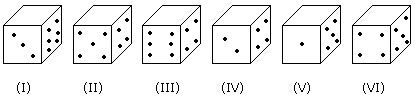The sum of the numbers of dots on the opposite face is 7. Question - If the odd numbered dice have even number of dots on their top faces, then what would be the total number of dots on the top faces of their dice?

Detailed Solution for Test: Dice- 2 - Question 1

Odd numbered dice are : (I), (III) and (V)

No. of dots on the top faces of these dice are 2, 2 and 4 respectively.

Required total = 2 + 2 + 4 = 8

Test: Dice- 2 - Question 2

### Directions to Solve Six dice with upper faces erased are as shows.The sum of the numbers of dots on the opposite face is 7. Question - If even numbered dice have even number of dots on their top faces, then what would be the total number of dots on the top faces of their dice?

Detailed Solution for Test: Dice- 2 - Question 2

Even numbered dice are: (II), (IV) and (VI)

No. of dots on the top face of (II) dice = 6

No. of dots on the top face of (IV) dice = 6

and No. of dots on the top face of (VI) dice = 6

Therefore Required total = 6 + 6 + 6 = 18

Test: Dice- 2 - Question 3

### Directions to Solve Six dice with upper faces erased are as shows.The sum of the numbers of dots on the opposite face is 7. Question - If dice (I), (II) and (III) have even number of dots on their bottom faces and the dice (IV), (V) and (VI) have odd number of dots on their top faces, then what would be the difference in the total number of top faces between there two sets?

Detailed Solution for Test: Dice- 2 - Question 3

No. of faces on the top faces of the dice (I), (II) and (III) are 5, 1 and 5 respectively.

Therefore, Total of these numbers = 5 + 1 + 5 = 11

No. of dots on the top faces of the dice (IV), (V) and (VI) are 1, 3 and 1 respectively.

Therefore, Total of these numbers = 1 + 3 + 1 = 5

Required difference = 11 - 5 = 6

Test: Dice- 2 - Question 4

Directions to Solve

Six dice with upper faces erased are as shows.The sum of the numbers of dots on the opposite face is 7.

Question -

If the even numbers of dice have odd number of dots on their top faces and odd numbered dice have even of dots on their bottom faces, then what would be the total number of dots on their top faces?

Detailed Solution for Test: Dice- 2 - Question 4

No. of dots on the top faces of the dice (II), (IV) and (VI) are 1, 1 and 1 respectively.

No. of dots on the top faces of the dice (I), (III) and (V) are 5, 5 and 3 respectively.

Required total = 5 + 5 + 3 + 1 + 1 + 1 = 16

Test: Dice- 2 - Question 5

Directions to Solve

Six dice with upper faces erased are as shows.The sum of the numbers of dots on the opposite face is 7.

Question -

If the dice (I), (II) and (III) have even number of dots on their bottom faces, then what would be the total number of dots on their top faces?

Detailed Solution for Test: Dice- 2 - Question 5

No. of dots on the top faces of dice (I), (II) and (III) are 5, 1 and 5 respectively.

Required total = 5 + 1 + 5 = 11

Test: Dice- 2 - Question 6

Directions to Solve

The figure given on the left hand side in each of the following questions is folded to form a box. Choose from the alternatives (1), (2), (3) and (4) the boxes that is similar to the box formed.

Question -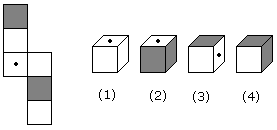Detailed Solution for Test: Dice- 2 - Question 6

As, shown in figure, these are opposite of each other. Thus, option (2) is correct.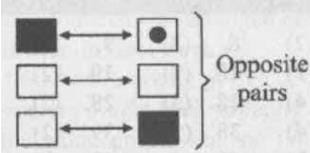Test: Dice- 2 - Question 7

Directions to Solve

The figure given on the left hand side in each of the following questions is folded to form a box. Choose from the alternatives (1), (2), (3) and (4) the boxes that is similar to the box formed.

Question -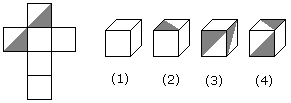Test: Dice- 2 - Question 8

Directions to Solve

The figure given on the left hand side in each of the following questions is folded to form a box. Choose from the alternatives (1), (2), (3) and (4) the boxes that is similar to the box formed.

Question -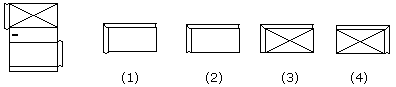Detailed Solution for Test: Dice- 2 - Question 8

The pattern on fig. (X) and also the fact that the faces are rectangle, indicate that only fig. (1) can be obtained by folding fig. (X).

Test: Dice- 2 - Question 9

Directions to Solve

The figure given on the left hand side in each of the following questions is folded to form a box. Choose from the alternatives (1), (2), (3) and (4) the boxes that is similar to the box formed.

Question -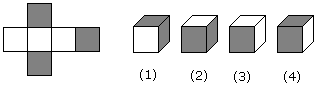Test: Dice- 2 - Question 10

Directions to Solve

The figure given on the left hand side in each of the following questions is folded to form a box. Choose from the alternatives (1), (2), (3) and (4) the boxes that is similar to the box formed.

Question -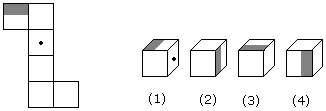## CSAT Preparation for UPSC CSE

72 videos|64 docs|92 tests
 Use Code STAYHOME200 and get INR 200 additional OFF Use Coupon Code
Information about Test: Dice- 2 Page
In this test you can find the Exam questions for Test: Dice- 2 solved & explained in the simplest way possible. Besides giving Questions and answers for Test: Dice- 2, EduRev gives you an ample number of Online tests for practice

## CSAT Preparation for UPSC CSE

72 videos|64 docs|92 tests